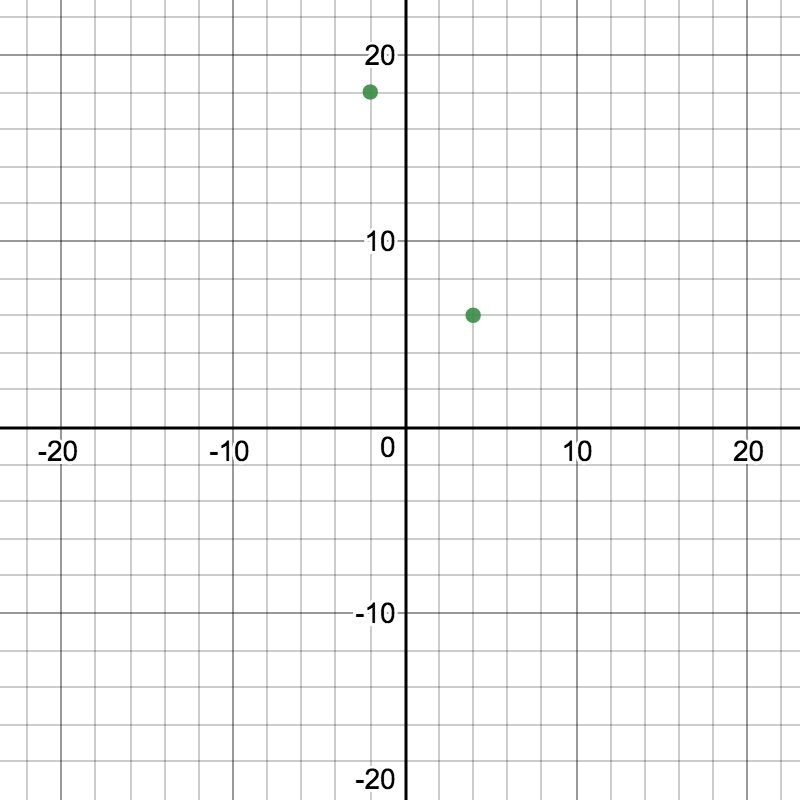# Writing Equations of Lines

1. The drama department sold adult and child tickets to a play and earned a total of $$25,700$$.  Adult tickets sold for $$35$$ and child tickets sold for $$18$$.
• Write an equation, in Standard Form, to represent the total revenue where $$x$$ represents the number of adult tickets sold and $$y$$ represents the number of child tickets sold.
• Convert your equation to Slope-Intercept Form.

1. Tomato plants were $$3$$ inches tall when purchased.  In order to ensure that the enclosure would be tall  enough, the plants were measured every few days so that they could estimate the height of the plants for 60 days.  The table below represents the number of days after planting and the corresponding height of the plant in inches.
 Days after planting $$(x)$$ Height of plant  in inches $$(y)$$ $$2$$ $$4$$ $$5$$ $$5\Large\frac{1}{2}$$ $$8$$ $$7$$ $$12$$ $$9$$
• Write the equation, in Slope-Intercept Form, that represents the growth of the plants given the number of days.
• If the enclosure is $$45$$ inches tall, will the plant fit inside this enclosure on day $$60$$?

1. Write the equation of the line, in Standard Form, between the two points shown in the graph below.